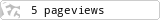通过javascript把图片转化为字符画1.获取上传图片对象数据

• Internet Explorer: 10+
• Firefox: 10+
• Chrome: 13+
• Opera: 12+
• Safari: partial

2.获取图像对象像素点

1. var imgData = c.getImageData(0, 0, img.width, img.height);
2. var imgDataArr = imgData.data;
3. var imgDataWidth = imgData.width;
4. var imgDataHeight = imgData.height;
5. for (h = 0; h < imgDataHeight; h += 12) {
6.     for (w = 0; w < imgDataWidth; w += 6) {
7.         var index = (w + imgDataWidth * h) * 4;
8.         var r = imgDataArr[index + 0];
9.         var g = imgDataArr[index + 1];
10.         var b = imgDataArr[index + 2];
11.     }
12. }

3.根据rgb值计算灰度

1、简化 sRGB IEC61966-2.1 [gamma=2.20]
Gray = (R^2.2 * 0.2126  + G^2.2  * 0.7152  + B^2.2  * 0.0722)^(1/2.2)
Gray = (R^2.2 * 0.2973  + G^2.2  * 0.6274  + B^2.2  * 0.0753)^(1/2.2)
3、Apple RGB [gamma=1.80]
Gray = (R^1.8 * 0.2446  + G^1.8  * 0.6720  + B^1.8  * 0.0833)^(1/1.8)
4、ColorMatch RGB [gamma=1.8]
Gray = (R^1.8 * 0.2750  + G^1.8  * 0.6581  + B^1.8  * 0.0670)^(1/1.8)
5、简化 KODAK DC Series Digital Camera [gamma=2.2]
Gray = (R^2.2 * 0.2229  + G^2.2  * 0.7175  + B^2.2  * 0.0595)^(1/2.2)

1. // 根据rgb值计算灰度
2. function getGray(r, g, b) {
3.     return 0.299 * r + 0.578 * g + 0.114 * b;
4. }

4.根据灰度生成相应字符

1. // 根据灰度生成相应字符
2. function toText(g) {
3.     if (g <= 30) {
4.         return ’8′;
5.     } else if (g > 30 && g <= 60) {
6.         return ’&’;
7.     } else if (g > 60 && g <= 120) {
8.         return ’\$';
9.     }  else if (g > 120 && g <= 150) {
10.         return ’*';
11.     } else if (g > 150 && g <= 180) {
12.         return ’o';
13.     } else if (g > 180 && g <= 210) {
14.         return ’!';
15.     } else if (g > 210 && g <= 240) {
16.         return ’;';
17.     }  else {
18.         return ‘.’;
19.     }
20. }

PS:已经把这个demo整合到我博客的小工具里了（通过模板实现）去看看？

posted @ 2013-10-24 08:34 流浪老三 阅读(...) 评论(...) 编辑 收藏
Edit by  三叶草